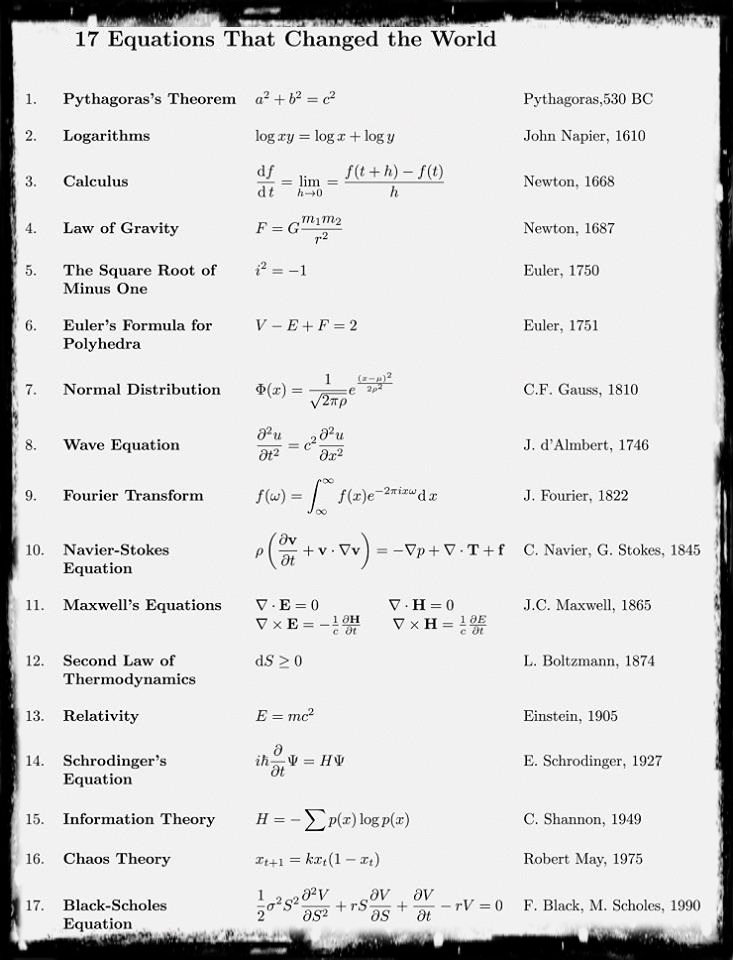Pythagorean Theorem- The Pythagorean Theorem was one of the earliest theorems known to ancient civilizations. This famous theorem is named for the Greek mathematician and philosopher, Pythagoras. Pythagoras founded the Pythagorean School of Mathematics in Cortona, a Greek seaport in Southern Italy. He is credited with many contributions to mathematics although some of them may have actually been the work of his students.

“The area of the square built upon the hypotenuse of a right triangle is equal to the sum of the areas of the squares upon the remaining sides.”

Logarithms-“Logarithm” is a word made up by Scottish mathematician John Napier (1550-1617), from the Greek word logos meaning “proportion, ratio or word” and arithmos meaning “number”, … which together makes “ratio-number” !

Calculus-Calculus is the mathematical study of change,in the same way that geometry is the study of shape and algebra
is the study of operations and their application to solving equations. It has two major branches, differential calculus , and
integral calculus ; these two branches are related to each other by the fundamental theorem of calculus.
Both branches make use of the fundamental notions of convergence of infinite sequences and infinite series to a well-defined limit. Generally considered to have been founded in the 17th century by Isaac Newton and Gottfried Leibniz.

law of gravity-Ã‚Â Newton’s law of universal gravitation states that any two bodies in the universe attract each other with a force that is directly proportional to the product of their masses and inversely proportional to the square of the distance between them. This is a general physical law derived from empirical observations by what Isaac Newton called induction.

The square root of minus 1-The imaginary unit or unit imaginary number, denoted as i, is a mathematical concept which extends the real number system Ã¢â€žÂÃ‚Â  to the complex number system Ã¢â€žâ€š, which in turn provides at least one root for every polynomial P(x), The imaginary unit’s core property is that i^2 = Ã¢Ë†â€™1. The term “imaginary” is used because there is no real number having a negative square.

Euler’s formula for polyhedra- V – E + F = 2.
Simple though it may look, this little formula encapsulates a fundamental property of those three-dimensional solids we call polyhedra, which have fascinated mathematicians for over 4000 years. Actually I can go further and say that Euler’s formula
tells us something very deep about shape and space. The formula bears the name of the famous Swiss mathematician Leonhard
Euler (1707 – 1783), who would have celebrated his 300th birthday this year.

Normal distribution-In probability theory, the normal (or Gaussian) distribution is a very commonly occurring continuous probability distributionÃ¢â‚¬â€a function that tells the probability that an observation in some context will fall between any two real numbers.

Wave equation-The wave equation is an important second-order linear partial differential equation for the description of waves Ã¢â‚¬â€œ as they occur in physics Ã¢â‚¬â€œ such as sound waves, light waves and water waves. the problem of a vibrating string such as that of a musical instrument was studied by Jean le Rond d’Alembert, Leonhard Euler, Daniel Bernoulli, and Joseph-Louis Lagrange. In 1746, dÃ¢â‚¬â„¢Alambert discovered the one-dimensional wave equation, and within ten years Euler discovered the three-dimensional wave equation.

Fourier transform- The Fourier transformÃ‚Â  named after Joseph Fourier, is a mathematical transformation employed to transform signals between time (or spatial) domain and frequency domain, which has many applications in physics and engineering. It is reversible, being able to transform from either domain to the other. The term itself refers to both the transform operation and to the function it produces.

Navier stokes equation- In physics, the NavierÃ¢â‚¬â€œStokes equations , named after Claude-Louis Navier and George Gabriel Stokes, describe the motion of fluid substances. These equations arise from applying Newton’s second law to fluid motion, together with the assumption that the stress in the fluid is the sum of a diffusing viscous term (proportional to the gradient of velocity) and a pressure term.

Maxwell’s equations– It represent one of the most elegant and concise ways to state the fundamentals of electricity and magnetism. From them one can develop most of the working relationships in the field. Because of their concise statement, they embody a high level of mathematical sophistication and are therefore not generally introduced in an introductory treatment of the subject, except perhaps as summary relationships.

Second law of thermodynamics-Ã‚Â The second law of thermodynamics states that the entropy of an isolated system never decreases, because isolated systems always evolve toward thermodynamic equilibriumÃ¢â‚¬â€ a state depending on the maximum entropy.

Relativity– Einstein’s theory of special relativity includes electricity and magnetism in a simple, logical extension of the relativity of Galileo and Newton. Its conclusions, including time dilation, length contraction, and E=mc2 have changed profoundly our ideas of time and space, matter and energy.

Ã‚Â Schrodinger equation The Schrodinger equation is the fundamental equation of physics for describing quantum mechanical behavior. It is also often called the Schrodinger wave equation, and is a partial differential equation that describes how the wave function of a physical system evolves over time.

Information theory Information theory is a branch of applied mathematics, electrical engineering, and computer science involving the quantification of information. Information theory was developed by Claude E. Shannon to find fundamental limits on signal processing operations such as compressing data and on reliably storing and communicating data.

Chaos Theory-Chaos theory is a field of study in mathematics, with applications in several disciplines including meteorology, physics, engineering, economics, biology, and philosophy. Chaos theory studies the behavior of dynamical systems that are highly sensitive to initial conditions

Black-scholes Equation- The BlackÃ¢â‚¬â€œScholes or BlackÃ¢â‚¬â€œScholesÃ¢â‚¬â€œMerton model is a mathematical model of a financial market containing certain derivative investment instruments. From the model, one can deduce the BlackÃ¢â‚¬â€œScholes formula,which gives a theoretical estimate of the price of European-style options